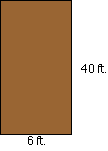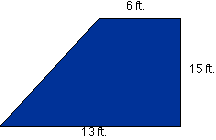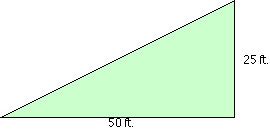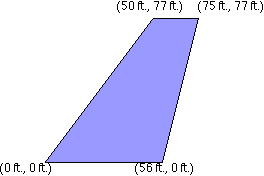PRINT THIS ANSWER SHEET TO FILL OUT AND TURN IN.

NAME_________________________________ CLASS____________________ DATE____________

## Wing Area (Level 2) Worksheet

USE INTEGRATION TO SOLVE THESE PROBLEMS.

YOU MAY FIND IT HELPFUL TO ROTATE SOME SHAPES.

1.  What is the area of the Wright Brother's rectangular planform?area = _______________

2. What is the area of the F-18 (Blue Angels) trapezoidal planform?area = _______________

3. What is the area of the Concorde triangular planform? (Note: These are not the actual dimensions.)area = _______________

4. What is the area of the Space Shuttle's compound planform? (Note: These are not the actual dimensions.)area = _______________

5. What is the area of the Boeing 747 trapezoidal planform? (Note: The coordinates of the vertices are given in the diagram. These are not the actual dimensions. Hint: Rotate the figure 90o or integrate along the y-axis with the equations of the lines solved for x as a function of y.)area = _______________

6. Design your own wing below including its dimensions.

7. What is the area of your airplane's planform?

8. Explain why you designed your planform the way you did.